# Option B: Engineering Physics (HL)

See the guide for this topic.

## B.3 – Fluids and fluid dynamics

• ### Density and pressure

Density may be defined as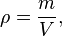where ρ is density, m is mass, and V is volume.

Pressure may be defined as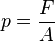where P is pressure, F is the force applied, and A is the area of the object in which the force is applied upon.This equation can be applied to any static fluid in any shaped container, or no container. The equation shows that, for a given fluid, the pressure depends only on depth (assuming we are referring only to locations where g is constant).

Thus,• ### Buoyancy and Archimedes’ principleThus, when an object is wholly or partially immersed in a fluid, it experiences an upthrust (buoyancy force) equal to the weight of the fluid displaced. This is Archimedes’ principle.• ### Pascal’s principle

Because any liquid is incompressible (its volume cannot be reduced) and its molecular motions are random, we can state that a pressure exerted anywhere in an enclosed static liquid will be transferred equally to all other parts of the liquid.

If different parts of the liquid are at different heights, however, this will result in additional differences in pressure, which may or may not be significant. This is the basis of hydraulic machinery.• ### Hydrostatic equilibrium

A fluid is in hydrostatic equilibrium if it is either at rest or if any parts of it that are moving have a constant velocity.

This will occur when forces are balanced by differences in pressure. For example:

• A floating boat will be in a state of hydrostatic equilibrium if it is balanced by pressure differences in the water.
• The Earth’s atmosphere (as a whole) is in hydrostatic equilibrium because pressure differences cross the atmosphere are balancing the effects of gravity on the air.
• Most stars are in hydrostatic equilibrium because the inwards gravitational attraction between the particles is opposed by the outwards pressure of the hot gases and radiation.

• ### The ideal fluid

We can idealize and simplify the flow of a fluid as the movement of layers sliding over each other (like playing cards sliding over each other), without any movement of fluid between those layers. This is described as laminar flow.Thus, an ideal fluid

• has constant density and is incompressible
• has constant pressure, acting equally in all directions
• is non-viscous meaning that it has no frictional forces opposing motion. There are no shear forces between layers, or frictional forces between layers or any surfaces with which they may come in contact. (Shear forces are non-aligned parallel forces that tend to push a substance in opposite directions.)
• has a steady flow pattern that does not change with time and which can be represented by streamlines.

• ### Streamlines

• Streamlines are lines that show the paths that (mass-less) objects would follow if they were placed in the flow of a fluid. The arrows in the picture above (in the previous section) represent streamlines.
• A tangent to a streamline shows the velocity of flow at that point.
• Streamlines cannot cross over each other.
• If the streamlines get closer together, the fluid must be flowing faster.
• Smoke or dye are often used in labs to mark the streamlines.

• ### The continuity equation

The mass per second entering and leaving the tube must be constant and, because the fluid is incompressible, the flow speed must increase where the tube’s cross-sectional area decreases.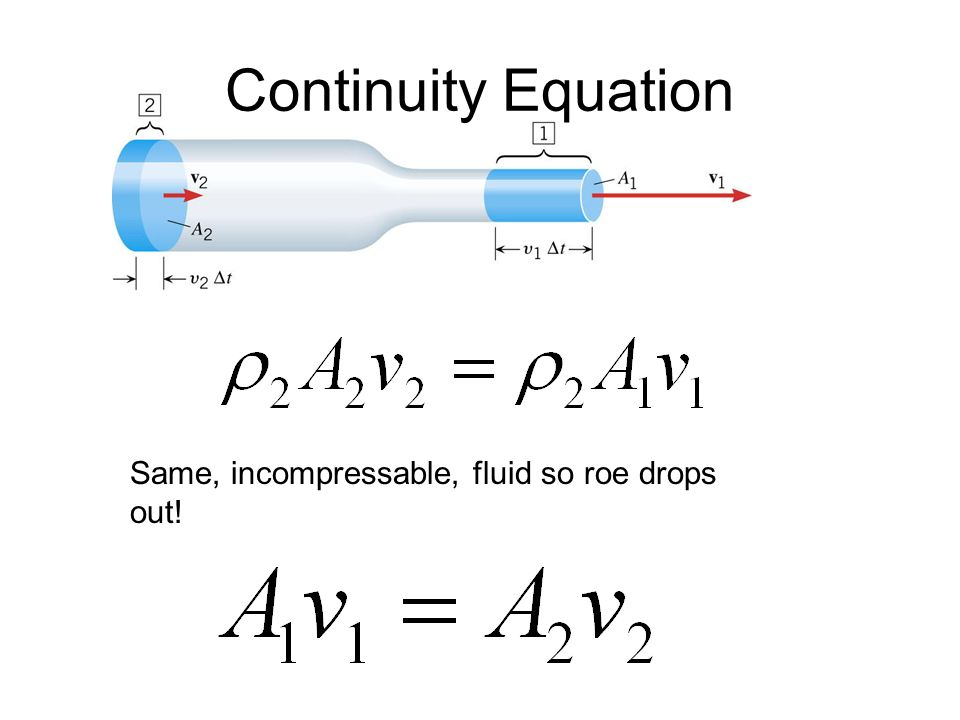• ### The Bernoulli equation and the Bernoulli effect

The Bernoulli equation

In general, we would expect that the speed of flow of an incompressible fluid in an enclosed system would increase if

• some kind of pump was providing a pressure difference
• the pipe was going down to a lower level
• the pipe was getting narrower.

The Bernoulli equation involves these factors in an equation that describes the steady flow of an ideal fluid in any system and may be given by(h is represented by z in the formula booklet)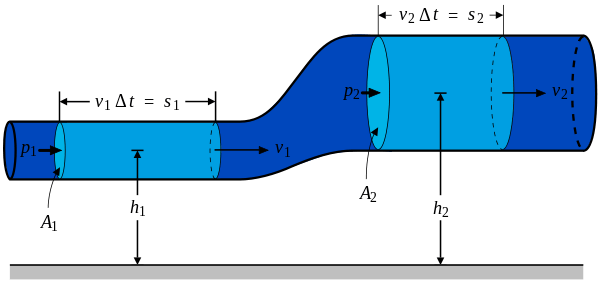The Bernoulli effect

For a fluid flowing horizontally (or with insignificant height variations), the Bernoulli equation reduces to 1/2ρv^2 + p = constant.

This shows us that if an (ideal) fluid is flowing horizontally, or an object is moving horizontally through a fluid without turbulence, there must be a decrease in pressure wherever the speed increases.

This is commonly known as the Bernoulli effect and it has many interesting applications such as

• The curved path of spinning balls – The motion of the ball’s surface will increase the speed of the air flow on one side and decrease it on the other. This effect will be greater if the surface of the ball is not smooth. The difference in air speeds causes a pressure difference and a force in one direction.• Aircraft wings – The cross-sectional shape of an aircraft wing (called its aerofoil or airfoil) will affect the way in which the air flows past it. If the shape causes the streamlines to be closer together above the wing, this increases the speed of the air and reduces the pressure, causing an upwards force called lift. The effect may be increased by raising the leading edge of the aerofoil; this also causes the force of the air striking the aerofoil to have a vertical component, increasing lift.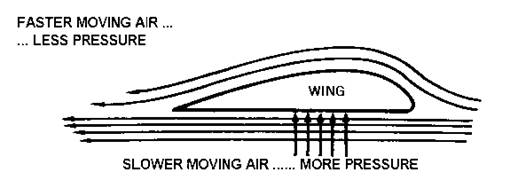• Venturi tubes – A fluid flowing through a tube will have less pressure at a place where the tube is narrower because the fluid must flow faster. Thus, measuring the decrease in pressure caused by a Venturi tube can be used to determine a fluid’s flow rate.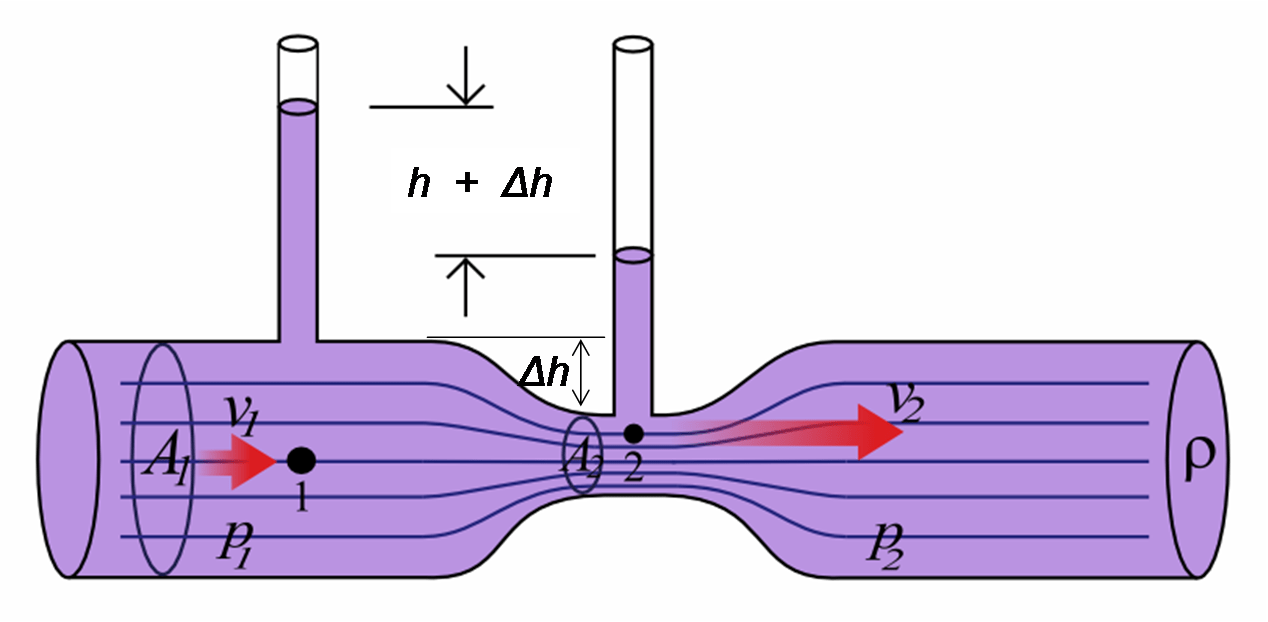The Venturi effect can be very useful in situations where fluids need to be mixed. A narrowing in a tube with one fluid flowing though it can produce a decrease in pressure that encourages another fluid to flow into the tube. For example, this is used in car engines to mix air and gasoline (petrol).

• ### Stokes’ law and viscosity

Viscosity

Contrary to our assumptions of an ideal fluid, no liquid is perfectly ideal because there will always be some frictional forces between different layers and the outer layers and any container. Thus, viscosity can be considered as a measure of a fluid’s resistance to flow.

Stokes’ law

When an object moves through a fluid it will experience a resistive force because of the viscosity of the fluid. This force is known as viscous drag. Stokes’s law provides a way of calculating the size of this drag force given that

• there must be streamline (laminar) flow
• spherical objects are used as test objects (drag force experienced by spheres)
• the test objects have smooth surfaces.where F is the drag force experienced by the test object (sphere), η is the coefficient of viscosity (different for different fluids), r is the radius of the sphere, and v is the velocity in which the test objects travels.

• ### Laminar and turbulent flow and the Reynolds number

• For laminar and turbulent flow, see previous section (The ideal fluid).
• As the mean speed, v, of a fluid through a pipe of radius r increases, laminar flow becomes less likely and turbulence may begin. The Reynolds number, R, is used as a guide to predict the conditions under which turbulent (non-laminar) flow will begin which may be given bywhere v is the mean speed of a fluid through a pipe, r is the radius of the pipe, ρ is the density of the fluid, and η is the coefficient of viscosity of the fluid.

• This equation demonstrates that a fluid can flow faster without causing turbulence if it has a larger viscosity and smaller density, and if larger dimensions are involved. The same equation can be used for estimating the maximum speed of an object of dimension r through a stationary fluid before turbulence will begin.
• Turbulence is often characterized by swirling currents, involving some flow in the opposite direction to most of the fluid. These are known as vortices and eddies. The alternate formation of vortices can result in oscillation forces on an object situated in a fluid flow, which can give rise to resonance effects.
• According to the syllabus, values where R<10^3 represent conditions for laminar flow.

## B.4 – Forced vibrations and resonance

• ### Natural frequency of vibration

• When something is disturbed and then left to oscillate without further interference, it is said to oscillate at its natural frequency of (free) vibration.
• An object made of only one material in a simple shape, a tuning fork for example, may produce a single natural frequency, but objects with more complicated structures will have a range of natural frequencies although one frequency may dominate.

• ### Q-factor and damping

Damping

The motions of all objects have frictional forces of one kind or another acting against them. Frictional forces always act in the opposite direction to the instantaneous motion of an oscillator and result in a reduction of speed and the transfer of kinetic energy (and, consequently, potential energy). Therefore, as with all other mechanical systems, useful energy is transferred from the oscillator into the surroundings (dissipated) in the form of thermal energy and sound. Consequently, an oscillator will move at slower and slower speeds, and its successive amplitudes will decrease in size. This effect is called damping.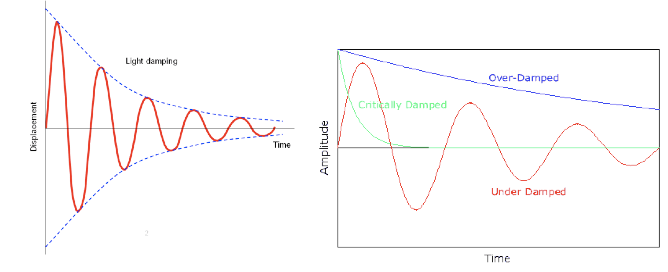There are various types of damping such as where

• some oscillations are over-damped because of considerable frictional forces. In effect no oscillations occur because resistive forces are such that the object takes a long time (compared to its natural period) to return to its equilibrium position. The decrease in amplitude with time is often exponential.
• conversely, occasionally damping can be very light and the oscillator may continue to oscillate, taking some time to dissipate its energy. A pendulum and a mass oscillating on a spring are good examples of under-damped systems. If the mass on the spring was placed in a beaker of oil (instead of air) it may then become over-damped.
• oscillations are often unhelpful or destructive and we may want to stop them as soon as possible. If an oscillation is stopped by resistive forces, such that it settles relatively quickly (compared to its natural period) back into its equilibrium position, without ever passing through it, the process is described as critically damped. A car’s suspension is an example of this kind of damping, as are self-closing doors.

Q-factor

We have seen that the amplitude, A, of a damped oscillator decreases approximately

exponentially with time. The Q (quality) – factor of an oscillator is a way of representing the degree of damping involved. A high Q-factor means that there is little damping.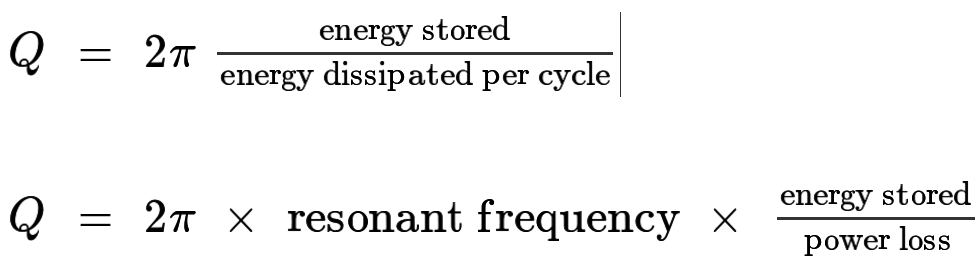where (one) cycle means (one) oscillation.

The Q factor for critical damping is usually quoted to be about 0.5, which suggests that most of the energy of the oscillator is dissipated in much less than one time period.

• ### Periodic stimulus and the driving frequency

• A forced oscillation occurs when an external oscillating force acts on another system tending to make it oscillate at a frequency that may be different from its natural frequency.
• The most important examples of forced oscillation are those in which the frequency of the external force (often called the driving frequency or the forcing frequency) is the same as the natural frequency. The child on the swing is an example of this. When a regular periodic stimulus to a system results in an increasing amplitude, the effect is called resonance.

• ### Resonance

Resonance is the increase in amplitude and energy of an oscillation that occurs when an external oscillating force has the same frequency as the natural frequency of the system. The oscillations of the driving force must be in phase with the natural oscillations of the system.

There are many important examples of resonance. Some are useful but many are unwanted and we usually try to reduce their damaging effects. Avoiding resonance in all kinds of structures is a major concern for engineers and it is an interesting combination of physics theory and practical engineering.

Some types of useful resonance may be where

• The molecules of certain gases in the atmosphere oscillate at the same frequency as infrared radiation emitted from the Earth. These gases absorb energy because of resonance; this results in the planet being warmer than it would be without the gases in the atmosphere. This is known as the greenhouse effect
• Radios and TVs around the world are ‘tuned’ by changing the frequency of an electronic circuit until it matches the driving frequency provided by the transmitted signal.
• Your legs can be thought of as pendulums with their own natural frequency. If you walk with your legs moving at that frequency, energy will be transferred more efficiently and it will be less tiring (we tend to do this without thinking about it).
• Quartz crystals can be made to resonate using electronics – the resulting oscillations are useful in driving accurate timing devices such as watches and computers.
• The sound from musical instruments can be amplified if the vibrations are passed on to a supporting structure that can resonate at the same frequency. An obvious example would be the strings on a guitar causing resonance in the box on which they are mounted. Because the box has a much larger surface area it produces a much louder sound than the string alone.
• Magnetic resonance imaging (MRI) is a widely used technique for obtaining images of features inside the human body. Electromagnetic waves of the right frequency (radio waves) are used to change the spin of protons (hydrogen nuclei) in water molecules.

Some types of unwanted resonance may be where

• Parts of almost all engines and machinery (and their surroundings) might vibrate destructively when their motors are operating at certain frequencies. For example, a washing machine may vibrate violently when the spinner is running at a certain frequency, and parts of vehicles can vibrate when the engine reaches a certain frequency, or they travel at certain speeds.
• Earthquakes may well affect some buildings more than others. The buildings that are most damaged are often those that have natural frequencies close to the frequencies of the earthquake.
• Strong winds or currents can cause dangerous resonance in structures such as bridges and towers. This is often due to the effect of eddies and vortices as the wind or water flows around the structure

To reduce the risk of damage from resonance, engineers can

• alter the shape of the structure to change the flow of the air or water past it.
• change the design so that the natural frequencies are not the same as any possible driving frequencies – this will involve changing the stiffness and mass of the relevant parts of the structure.
• ensure that there is enough damping in the structure and that it is not too rigid, so that energy can be dissipated.

### Referenced sources

• PHYSICS FOR THE IB DIPLOMA © CAMBRIDGE UNIVERSITY PRESS 2015
• Hodder Education Option B Chapter 14 – Engineering physics